StatLect

# Kullback-Leibler divergence

The Kullback-Leibler divergence is a measure of the dissimilarity between two probability distributions.## Definition

We are going to give two separate definitions of Kullback-Leibler (KL) divergence, one for discrete random variables and one for continuous variables.

Definition Letandbe two discrete random variables with supportsandand probability mass functionsand. Let, so thatThen the KL divergence offromisNote that the summation is over the support of, so that we always haveand, and, as a consequence, the natural logarithmis always well-defined.

The KL divergencemeasures how much the distribution defined byis dissimilar from the reference distribution defined by.

The definition for continuous random variables is analogous.

Definition Letandbe two continuous random variables with supportsandand probability density functionsandsuch that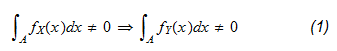for any set. Then the KL divergence offromisIn order to be entirely rigorous the above definition should also specify that the setsmust be measurable (see the lecture on probability for a definition of measurable set).

Property (1), which is called absolute continuity, requires that if the distribution associated to the densityassigns a non-zero probability to a set, then also the distributionmust assign a non-zero probability to that set. This requirement is analogous to that for discrete variables and ensures thatis well-defined on all sets that have non-zero probability.

## The KL divergence is non-negative

The next proposition states a fundamental property of the Kullback-Leibler divergence.

Proposition Letandbe two probability mass functions and. If the two probability mass functions coincide, that is, iffor all, thenOtherwise, if they do not coincide, thenProof

Let us first prove the equality part. If the two probability mass functions coincide, thenforand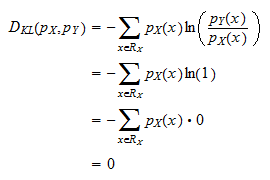When they do not coincide, then we have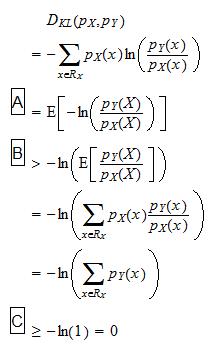where: in stepwe have written the summation as an expected value with respect to the probability distribution of; in step, we have used Jensen's inequality (the functionis strictly convex inand the random variableis not constant because the two probability mass function do not coincide); in stepwe have used the fact that a sum of probabilities cannot be greater than 1.

A similar result holds for continuous variables.

Proposition Letandbe two probability density functions such that their KL divergence is well-defined. If the two probability density function coincide almost surely, that is, if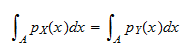for all measurable sets, thenOtherwise, if they do not coincide almost surely, thenProof

The proof is analogous to that for discrete variables.

## Asymmetry

An often cited property of the KL divergence is that it is not symmetric, that is, in general there is no guarantee that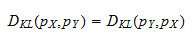In fact, it is even possible thatexists whenis not well-defined: as you can check by looking at the definition of KL divergence, this happens when the support ofis strictly included in the support of:## Why the KL divergence is a measure of dissimilarity

Since the Kullback-Leibler divergence is an information-theoretic concept and most of the students of probability and statistics are not familiar with information theory, they struggle to get an intuitive understanding of the reason why the KL divergence measures the dissimilarity of a probability distribution from a reference distribution. We provide an explanation that is entirely based on probabilistic concepts.

Suppose thatandare two probability mass functions such that the KL divergenceis well-defined.

Take a convex combination of the two distributions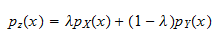where.

By increasingwe can makemore and more similar tountil, when,andcoincide.

It is possible to prove that the KL divergence is convex (see Cover and Thomas 2006) and, as a consequence,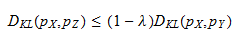Thus, the higheris, the smallerbecomes. In other words, the moreis similar to, the smaller the Kullback-Leibler divergence becomes.

## References

Cover, T. M., and J. A. Thomas (2006) " Elements of information theory", Wiley-Interscience.